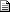# Maths - Projections of lines on planes - Forum Discussion 2

 By: Nobody/Anonymous - nobodyAddition to Projections (line on plane)   2004-07-11 19:04 I would like to make the following additions to the page. First of all, I would like to state that the plane's orientational vector should be a normal if it is to be used in the projection matrix equation: projection matrix = [I] - v * vt v should be the plane's normal. Further more I would like to add that a plane can also be described by it's two edge vectors. The vector describing it's orientation is then simply the cross product of those two vectors. This information might not add new information, since it can be distilled from the page as it is. However, it would have been very useful for me (a beginner) if I would have known about this. I ran into a problem because I used orientation vectors that weren't normals :(.
 By: Martin Baker - martinbakerRE: Addition to Projections (line on plane)   2004-07-12 09:34 Thanks very much for this, I will update the web pages as you suggest: about this page: https://www.euclideanspace.com/maths/geometry/elements/plane/lineOnPlane/ > projection matrix = [I] - v * vt The equations on this page seem to give: matrix for parallel component = v * vt matrix for perpendicular component = v * vt - [I] In other words my terms are all negative compared with your expression, have I made an error somewhere? Good point about the edge vectors, I will put this on the page defining the plane. I'll also try to make it clearer about the vectors being normals. Thanks for the feedback, MartinNew Foundations for Classical Mechanics (Fundamental Theories of Physics). This is very good on the geometric interpretation of this algebra. It has lots of insights into the mechanics of solid bodies. I still cant work out if the position, velocity, etc. of solid bodies can be represented by a 3D multivector or if 4 or 5D multivectors are required to represent translation and rotation.